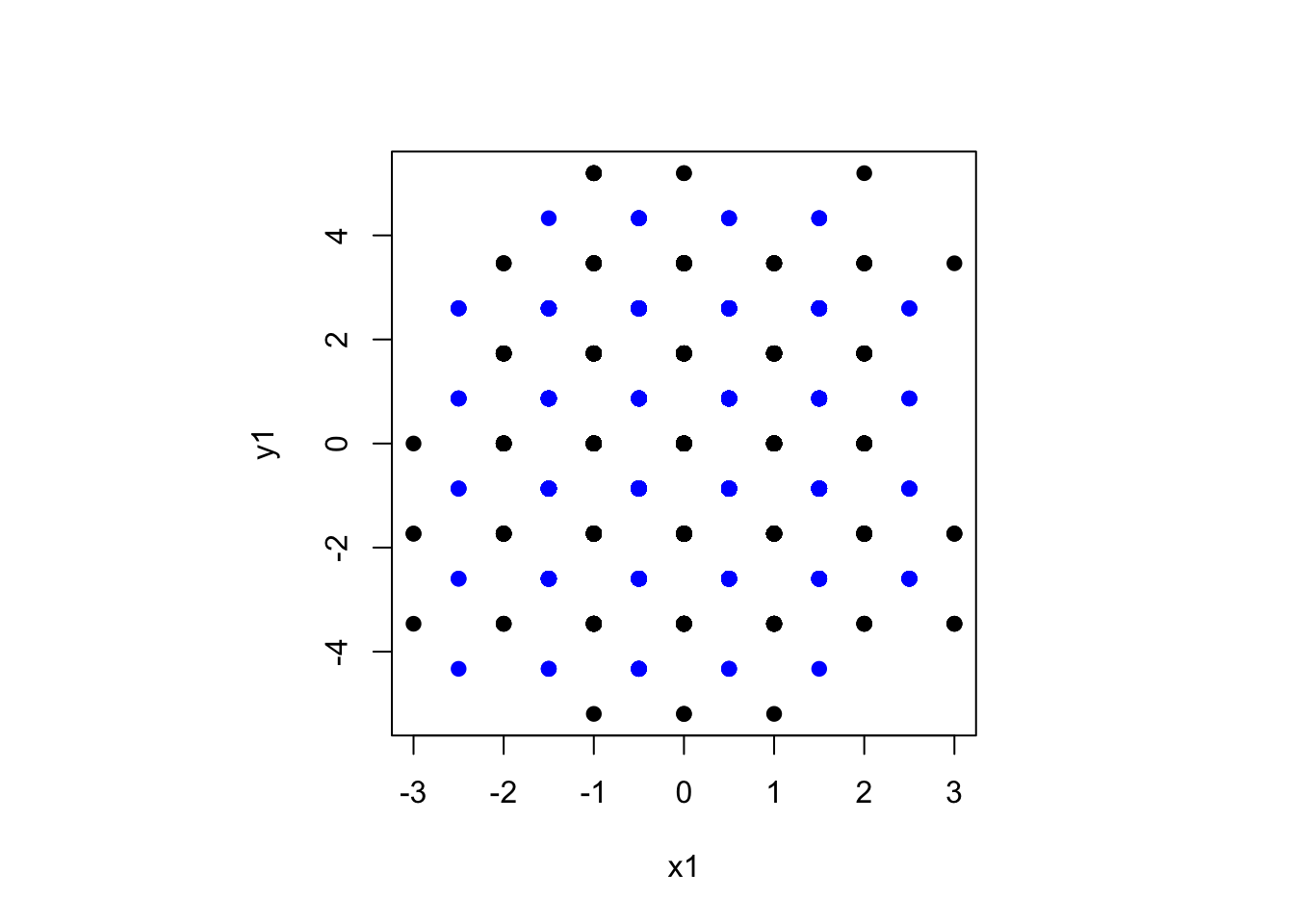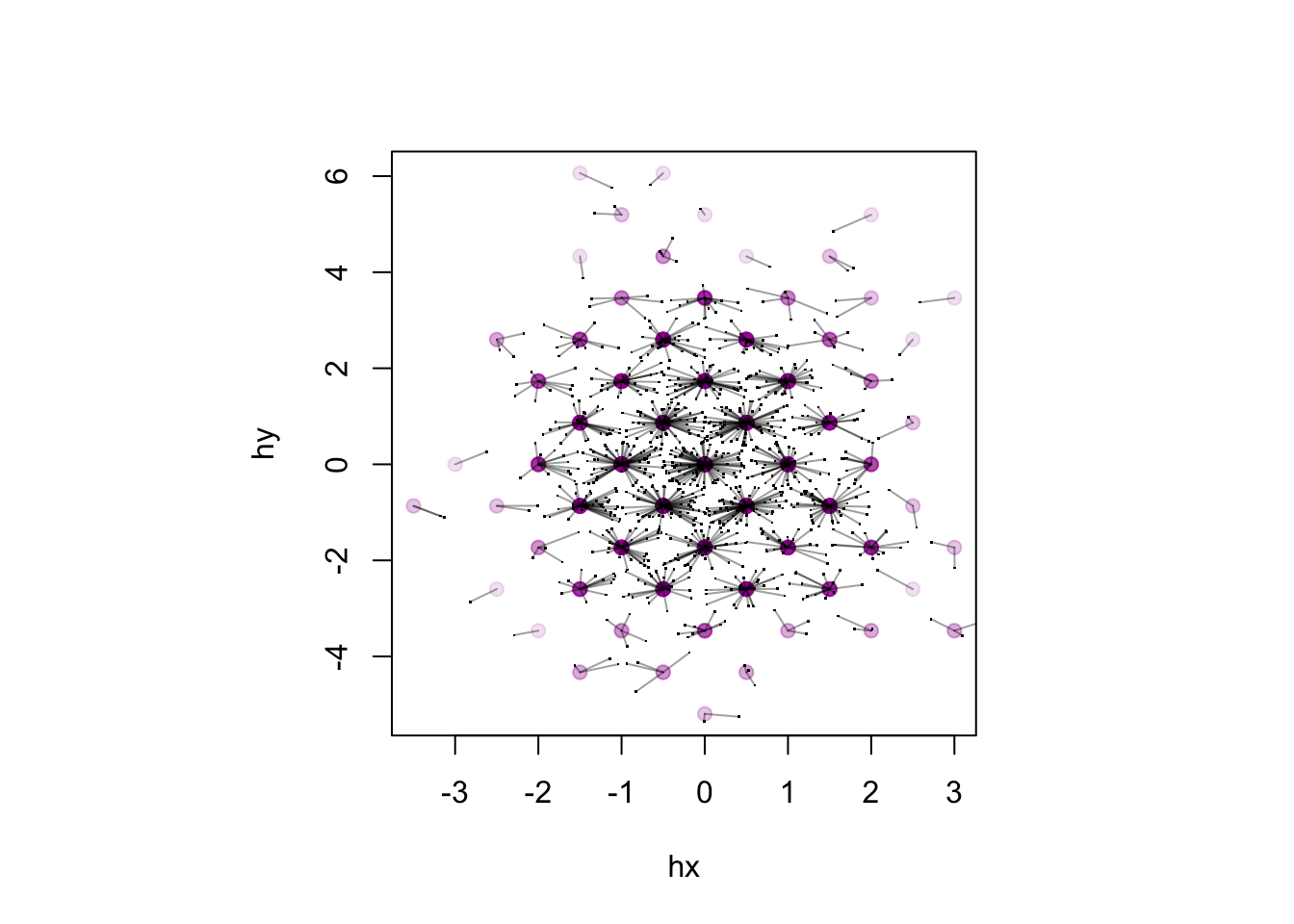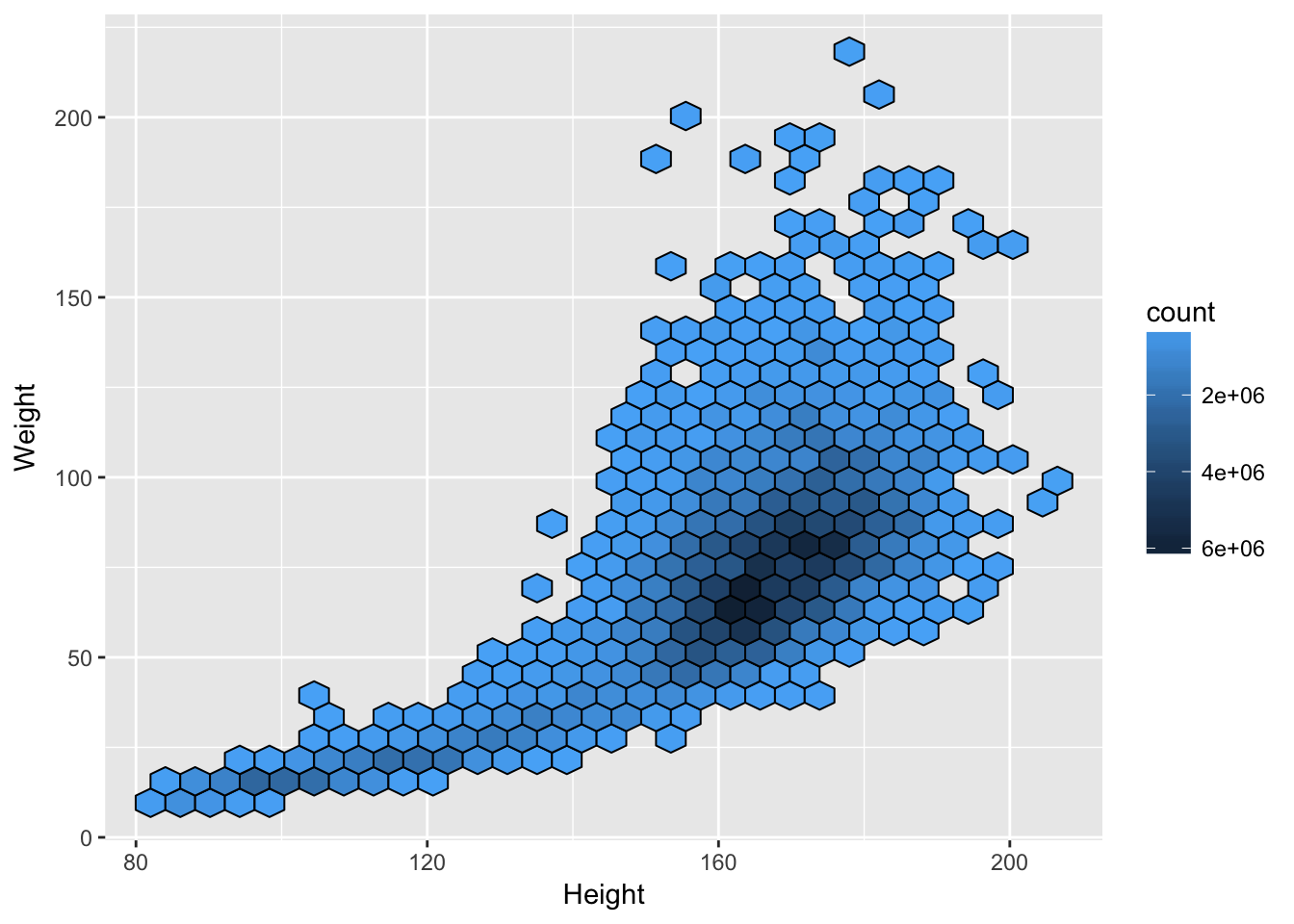# Survey Analysis in SQL and R

ToolsTools & LanguagesWorkflowposted by Thomas Lumley July 5, 2018

Charco Hui, as his Honours project in Statistics, has been writing a package for complex-survey analysis using dplyr and dbplyr. It’s here. At the moment...Charco Hui, as his Honours project in Statistics, has been writing a package for complex-survey analysis using `dplyr` and `dbplyr`. It’s here. At the moment it has only been tested with MonetDB, using the github version (0.5.2) of `MonetDBlite`, but it should work with many other databases (not SQLite, at the moment). I hope it’s still under development: the approach does seem to be useful for large survey data sets – and for smaller data sets the `dplyr` version is faster than the `survey` package, though more limited.

Mostly, the package does the same things as my old `sqlsurvey` package, just with `dbplyr` as middleware to avoid hand-coding all the SQL. One newish thing is the code for hexagonal binning. In `sqlsurvey` I did this by reading the data into memory in chunks, but that’s not necessary.

The algorithm for hexagonal binning isn’t new: you compute which bin the point would go into for two offset rectangular grids and take the closer of the two resulting bin centres as the centre of the hex.

Here’s a simplified version in R, bypassing the trivial but annoying centering and scaling you need to get away from (0,0), and not being quite careful enough about edge effects for outliers.

``````x<-rnorm(1000, sd=1)
y<-rnorm(1000, sd=sqrt(3))
x1<-round(x)
x2<-round(x+1/2)-1/2
y1<-round(y/sqrt(3))*sqrt(3)
y2<-(round(y/sqrt(3)+1/2)-1/2)*sqrt(3)
d1<-(x-x1)^2+3*(y-y1)^2
d2<-(x-x2)^2+3*(y-y2)^2
hx<-ifelse(d1<d2,x1,x2)
hy<-ifelse(d1<d2,y1,y2)
par(pty="s")
plot(x1,y1,pch=19)
points(x2,y2,pch=19,col="blue")````````````## now show how each point maps to a center
plot(hx,hy, col="#A000A020",pch=19)
points(x,y,pch=".")
segments(x,y,hx,hy,col="#00000060")``````You can do this in `dplyr`, of course. This code doesn’t plot, but it shows the aggregation of points to hexes that you’d need for plotting

``````library(dplyr, quietly=TRUE)
tibble(x,y) %>%
mutate(x1=round(x),y1=round(y/sqrt(3))*sqrt(3),
x2=round(x+1/2)-1/2, y2=(round(y/sqrt(3)+1/2)-1/2)*sqrt(3)) %>%
mutate(d1=(x-x1)^2+3*(y-y1)^2, d2=(x-x2)^2+3*(y-y2)^2) %>%
mutate(hx=if_else(d1<d2,x1,x2), hy=if_else(d1<d2,y1,y2)) %>%
group_by(hx,hy) %>% summarise(count=n())``````
``````## # A tibble: 65 x 3
## # Groups:   hx [?]
##       hx     hy count
##    <dbl>  <dbl> <int>
##  1  -3.5 -0.866     2
##  2  -3    0         1
##  3  -2.5 -2.60      1
##  4  -2.5 -0.866     2
##  5  -2.5  2.60      3
##  6  -2   -3.46      1
##  7  -2   -1.73      4
##  8  -2    0        12
##  9  -2    1.73      9
## 10  -1.5 -4.33      3
## # ... with 55 more rows``````

And once you’ve got in `dplyr`, you can just run it in `dbplyr` to do the computations in the database

For survey data, we want `summarise(count=sum(weights))` rather than `summarise(count=n())` so that the hex size depends on the estimated population size rather than the sample size, but otherwise it’s the same. That actually simplifies the edge-effect problem, as you can just add a point with zero weight at each potential hex center.

And here’s a small example,

``````# devtools:::install_github(chrk623/svydb)
library(svydb,quietly=TRUE)
library(DBI)
library(MonetDBLite)
con = dbConnect(MonetDBLite())

data(nhane)
dbWriteTable(con, "nhanes",nhane)``````
``## Identifier(s) "X", "SurveyYr", "ID", "Gender", "Age", "AgeMonths", "Race1", "Race3", "Education", "MaritalStatus", "HHIncome", "HHIncomeMid", "Poverty", "HomeRooms", "HomeOwn", "Work", "Weight", "Length", "HeadCirc", "Height", "BMI", "BMICatUnder20yrs", "BMI_WHO", "Pulse", "BPSysAve", "BPDiaAve", "BPSys1", "BPDia1", "BPSys2", "BPDia2", "BPSys3", "BPDia3", "Testosterone", "DirectChol", "TotChol", "UrineVol1", "UrineFlow1", "UrineVol2", "UrineFlow2", "Diabetes", "DiabetesAge", "HealthGen", "DaysPhysHlthBad", "DaysMentHlthBad", "LittleInterest", "Depressed", "nPregnancies", "nBabies", "Age1stBaby", "SleepHrsNight", "SleepTrouble", "PhysActive", "PhyActiveDays", "TVHrsDay", "ComputerHrsDay", "Alcohol12PlusYr", "AlcoholDay", "AlcoholYear", "SmokeNow", "Smoke100", "SmokeAge", "Marijuana", "AgeFirstMarij", "RegularMarij", "AgeRegMarij", "HardDrugs", "SexEver", "SexAge", "SexNumPartnLife", "SexNumPartYear", "SameSex", "SexOrientation", "WTINT2YR", "WTMEC2YR", "SDMVPSU", "SDMVSTRA" contain uppercase or reserved SQL characters and need(s) to be quoted in queries.``
``## Identifier(s) "Work" are reserved SQL keywords and need(s) to be quoted in queries.``
``````nhanes.db = tbl(con, "nhanes")
nh.dbsurv = svydbdesign(st = SDMVSTRA , wt = WTMEC2YR,id = SDMVPSU , data = nhanes.db)
hb = svydbhexbin(Weight~Height , design = nh.dbsurv)
svydbhexplot(hb, xlab = "Height", ylab = "Weight")``````Original Source## Thomas Lumley

Thomas Lumley attended Monash University (B.Sc.(Hons) in Pure Mathematics), the University of Oxford (M.Sc. in Applied Statistics) and the University of Washington, Seattle (PhD in Biostatistics). He spent twelve years on the faculty of the Department of Biostatistics at the University of Washington, and then moved to Auckland in 2010. He is still an Affiliate Professor at the University of Washington.

1